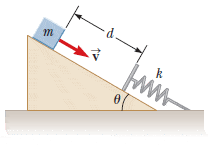# Relating to potential energy of a spring and mass on inclined plane

NewSoul

## Homework Statement

Here is an illustration to ease understanding:Angle inclined plane: θ = 20.0°
spring of force constant k = 525 N/m
block: m = 2.71 kg
distance of block from spring: d = 0.330 m
speed of block: v = 0.750 m/s

By what distance is the spring compressed when the block momentarily comes to rest? (I'm going to call it 'x' in this problem.)

The answer should be x = 0.143 m

## Homework Equations

Ok, I'm not quite sure how many of these are relevant or if I'm missing some, but I'll just give some that I know.

PEs = (1/2)kx2 (x = distance spring is compressed)
PEg = mgh
KE = (1/2)mv2
F = ma
U + KE = 0 (U = any potential energy? All potential energies? I'm not quite positive what is going on here.)

## The Attempt at a Solution

Okay. I'm not quite sure how to go about this, so I'll just start doing what I seem to know.

Let's start with force. It doesn't seem inherently relevant to the problem, but why not?

Okay, so force in the x direction seems to be: mgsinθ = ma. So we get a = gsinθ = (9.8)(sin 20°) = 3.35198 m/s2.

Force in the y direction seems to be: N - mgsinθ = 0. So N = mgsinθ = (2.71)(9.8)(sin 20°) = 9.08337 N.

-------------

Ok. That was probably not relevant. Let's do work now.

Using the numbers above, I get KE = (1/2)(2.71)(.750)2 = 0.76219 J.

PEg = (2.71)(9.8)h. Okay, I'm not quite sure if h should just be d or if I have to factor in the slope. I'm thinking that slope probably does have to be included, so h is 0.330(sin 20°), so PEg is 2.99751 J.

So it seems like PEg + PEs + KE = 0. Then it must be that (1/2)kx2 + mgh + (1/2)mv2 = 0... However, solving for x gives me the square root of a negative number, I must be interpreting U + KE = 0 wrong. Where do I go from here? Thanks, I know this is long.

nasu
Why do you think that the energy is zero?
You can use conservation of energy to solve the problem.
Equate the total energy in the initial position with the total energy in the final position.
Total energy here includes kinetic, potential gravitational and potential elastic.
Some terms may be zero, like elastic energy in the initial position or kinetic energy in the final position.

NewSoul
Why do you think that the energy is zero?
Well, I'm not entirely sure what is meant by the equation U + KE = 0 or if it's relevant...But I do know that a decrease in potential energy would mean an increase in kinetic energy and vice versa.

You can use conservation of energy to solve the problem.
Equate the total energy in the initial position with the total energy in the final position.
Total energy here includes kinetic, potential gravitational and potential elastic.
Some terms may be zero, like elastic energy in the initial position or kinetic energy in the final position.

So are you saying that PEg = PEs (KE is zero at the initial and final positions)? I'm afraid I don't understand.

*edit* Hold on a minute I did something weird...

Okay, so if PEg = PEs, then I get (1/2)kx2 = mgh... and x = √(2mgh/k) = 0.107 m, which is incorrect. If I assume h shouldn't have sin in it, then I get x = 0.183 m. Both of these answers are still wrong. Shouldn't I have to fit velocity into the problem somehow?

Last edited:
Homework Helper
Gold Member
Okay, so if PEg = PEs, then I get (1/2)kx2 = mgh... and x = √(2mgh/k) = 0.107 m, which is incorrect. If I assume h shouldn't have sin in it, then I get x = 0.183 m. Both of these answers are still wrong. Shouldn't I have to fit velocity into the problem somehow?
h is the total vertical movement of the block. Don't forget that this will include a contribution from x.

nasu
So are you saying that PEg = PEs (KE is zero at the initial and final positions)? I'm afraid I don't understand.

*edit* Hold on a minute I did something weird...

Okay, so if PEg = PEs, then I get (1/2)kx2 = mgh... and x = √(2mgh/k) = 0.107 m, which is incorrect. If I assume h shouldn't have sin in it, then I get x = 0.183 m. Both of these answers are still wrong. Shouldn't I have to fit velocity into the problem somehow?

No, the initial KE is not zero. It moves with some speed, isn't it?
And h is measured along the vertical direction.